# “Circle Formulas” for Electronics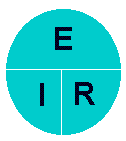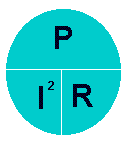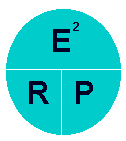## Power

E=Voltage in Volts
I=Current in Amps
R=Resistance in Ohms
P=Power in Watts
I=Current in Amps
E=Voltage in Volts
E=Voltage in Volts
R=Resistance in Ohms
P=Power in Watts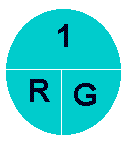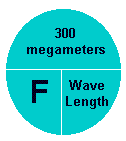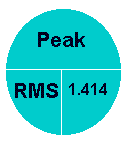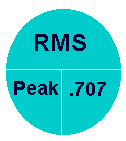## AC Voltage

R=Resistance in Ohms
G=Conductance in Mhos
F=Frequency in Hertz
Wavelength in Meters
RMS=Root Mean Square value
Peak=Peak voltage in a sine wave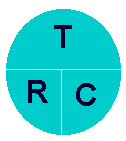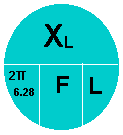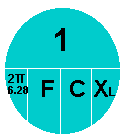## Capacitive Reactance

T=Time in Seconds
R=Resistance in Ohms
T=Time in Seconds
F=Frequency in Hertz
Xl=Inductive Reactance in Ohms
F=Frequency in Hertz
L=Impedance in Henrys
F=Frequency in Hertz
Xc=Capacitive Reactance in Ohms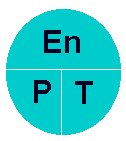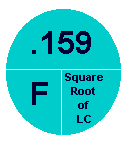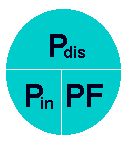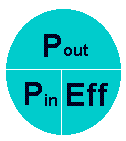## Efficiency

En=Energy
P=Power in Watts
T=Time in Seconds
F=Frequency in Hertz Pdis=Power Dissipated in Watts
Pin=Power in in Watts
PF=Power Factor
Pout=Power out in Watts
Pin=Power in in Watts
Eff=Efficiency